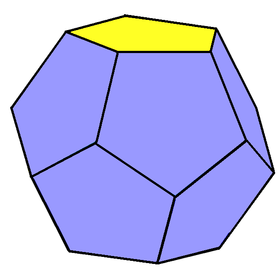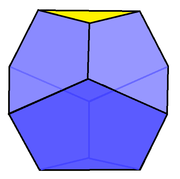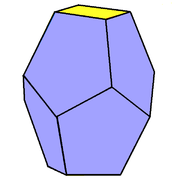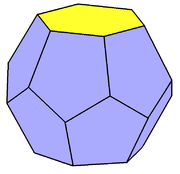# Truncated trapezohedron

Set of truncated trapezohedraFaces2 n-gons,
2n pentagons
Edges6n
Vertices4n
Symmetry groupDnd, [2+,2n], (2*n), order 4n
Rotation groupDn, [2,n]+, (22n), order 2n
Dual polyhedrongyroelongated dipyramids
Propertiesconvex

An n-gonal truncated trapezohedron is a polyhedron formed by a n-gonal trapezohedron with n-gonal pyramids truncated from its two polar axis vertices. If the polar vertices are completely truncated (diminished), a trapezohedron becomes an antiprism.

The vertices exist as 4 n-gons in four parallel planes, with alternating orientation in the middle creating the pentagons.

The regular dodecahedron is the most common polyhedron in this class, being a platonic solid, with 12 congruent pentagonal faces.

A truncated trapezohedron has all vertices with 3 faces. This means that the dual polyhedra, the set of gyroelongated dipyramids, have all triangular faces. For example, the icosahedron is the dual of the dodecahedron.

## Forms